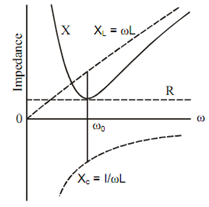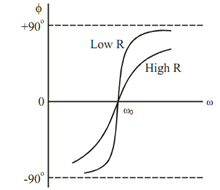## Impedance Curve Assignment Help

Assignment Help: >> Series and Parallel Resonance - Impedance Curve

Impedance Curve:

Figure illustrates variation of impedance Z, inductive reactance XL and capacitive reactance XC with frequency. At resonance XL = XC and Z = R.Figure: Impedance Curve

Angle φ (between voltage and current) is given by

φ= tan-1  ( X L - X c ) /R

Here, φ is known as the power factor angle. Figure illustrates the variation of φ with frequency.

At frequencies below ω0 the capacitive reactance is greater than the inductive reactance and the angle of the impedance is -ve. If the resistance is low, the angle alters more quickly with frequency as illustrated in Figure. As ω approaches zero the angle of Z approaches - 90.Figure: Angle Curve